Click to Chat

1800-1023-196

+91-120-4616500

CART 0

• 0

MY CART (5)

Use Coupon: CART20 and get 20% off on all online Study Material

ITEM
DETAILS
MRP
DISCOUNT
FINAL PRICE
Total Price: Rs.

There are no items in this cart.
Continue ShoppingChemical Formulas

Table of Content

Formula of Empirical

Formula of Molecular

Solved Examples on Empirical formula  and Molecular Formula

Related Resources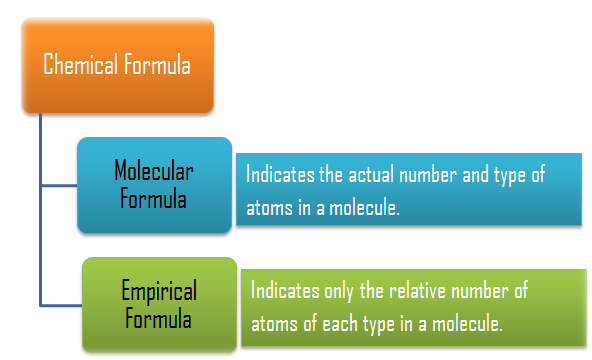Formula of Empirical

It is the formula which expresses the smallest whole number ratio of the constituent atom within the molecule.
Empirical formula of different compound may be same.So it may or may not represent the actual formula of the molecule.
It can be deduced by knowing the weight % of all the constituent element with their atomic masses for the given compound.

For example: C6H12O6, CH3COOH, HCHO

All have same empirical formula CH2O, but they are different.

The empirical formula of a compound can be determined by the following steps:

Write the name of detected elements in column-1 present in the compound.

Write the corresponding atomic mass in column-2.

Write the experimentally determined percentage composition by weight of each element present in the compound in column-3.

Divide the percentage of each element by its atomic weight to get the relative number of atoms of each element in column-4.

Divide each number obtained for the respective elements in step (3) by the smallest number among those numbers so as to get the simplest ratio in column-5.

If any number obtained in step (4) is not a whole number then multiply all the numbers by a suitable integer to get whole number ratio. This ratio will be the simplest ratio of the atoms of different elements present in the compound. Empirical formula of the compound can be written with the help of this ratio in column-6.

Refer to the following video for empirical formula

Formula of MolecularThe formula which represents the actual number of each individual atom in any molecule is known as molecular formula.

For certain compounds the molecular formula and the empirical formula may be same.

Molecular Formula = (Empirical Formula)n

Molecular Weight = Empirical formula weight x n

If the vapour density of the substance is known, its molecular weight can be calculated by using the equation.

2 x Vapour Density = Molecular weight

Solved Examples on Empirical formula  and Molecular Formula

Question 1:

A compound contains C = 71.23%, H = 12.95% and O = 15.81%. What is the empirical formula of the compound?

Solution

Element’s name with their symbol

Atomic mass

Weight % in compound

Relative number of atom

Simplest atomic ratio

Empirical formula

Carbon (C)

12

71.93Hydrogen (H)

1

12.95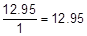Oxygen (O)

16

15.81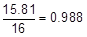Question 2:

The simplest formula of a compound containing 50% of element X (Atomic mass = 10) and 50% of the element Y (Atomic mass = 20) is:

a. XY

b. X2Y

c. XY2

d. X2Y3

Solution

Element’s name with their symbol

Atomic mass

Weight % in compound

Relative number of atom

Simplest atomic ratio

Empirical formula

X

10

50

50/10 = 5

5/2.5 = 2

X2Y

Y

20

50

50/20 = 2.5

2.5/2.5 = 1

Hence (B) is correct.

Question 3:

A compound of carbon, hydrogen and nitrogen contains these elements in the ratio 9:1:3.5. Calculate the empirical formula. If its molecular mass is 108, what is the molecular formula?

Solution:

Element

Element ratio

Atomic mass

Relative number of atoms

Simplest ratio

Carbon

9

12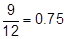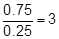Hydrogen

1

1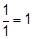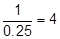Nitrogen

3.5

14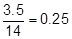Empirical formula = C3H4N

Empirical formula mass = (3 x 12) + (4 x 1) + 14 = 54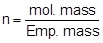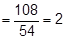Thus, molecular formula of the compound = 2 x empirical formula = 2 x C3H4N

= C6H8N2

Question 4:

2.38 gm of uranium was heated strongly in a current of air. The resulting oxide weighed 2.806 g. Determine the empirical formula of the oxide. (At. mass U = 238; O = 16).

Solution:
Step 1: To calculate the percentage of uranium and oxygen in the oxide.

2.806 g of the oxide contain uranium = 2.38 g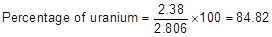Hence, the percentage of oxygen in the oxide = 100.00 – 84.82 = 15.18

Step 2: To calculate the empirical formula

Element

Symbol

Percentage of elements

At. mass of elements

Relative no. of atoms

=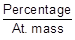Simplest atomic ratio

Simplest whole no. atomic ratio

Uranium

U

84.82

238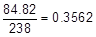3

Oxygen

O

15.18

16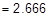8

Hence the empirical formula of the oxide is U3O8.

Question 5:

Chemical analysis of a carbon compound gave the following percentage composition by weight of the elements present. Carbon 10.06%, hydrogen 0.84%, chlorine 89.10%. Calculate the empirical formula of the compound.

Solution:
Step 1:   Percentage of the elements present

Carbon               Hydrogen                        Chlorine

10.06                     0.84                            89.10

Step 2:  Dividing the percentage compositions by the respective atomic weights of the elements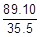0.84                           0.84                              2.51

Step 3:  Dividing each value in step 2 by the smallest number among them to get simple atomic ratio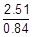Step 4:  Ratio of the atoms present in the molecule C  : H  : Cl

1  :  1  :   3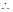The empirical formula of the compoundor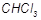.

Question 6:

A carbon compound on analysis gave the following percentage composition. Carbon 14.5%, hydrogen 1.8%, chlorine 64.46%, oxygen 19.24%. Calculate the empirical formula of the compound.

Solution:
Step1: Percentage composition of the elements present in the compound.

C    :                        H          :            Cl         :            O

14.5                         1.8                     64.46                 19.24

Step 2:  Dividing by the respective atomic weights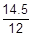: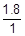: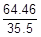1.21                          1.8                     1.81                     1.2

Step 3:  Dividing the values in step 2 among them by the smallest number.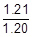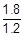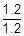Step 4:  Multiplication by a suitable integer to get whole number ratio.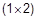2                               3                        3                      2The simplest ratio of the atoms of different elements in the compound.

C : H : Cl : O = 2 : 3 : 3 : 2Empirical formula of the compound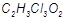.

Question 7:

The empirical formula of a compound is. Its molecular weight is 90. Calculate the molecular formula of the compound. (Atomic weights C = 12, H = 1, O = 16)

Solution:

Empirical formulaEmpirical formula weight = (12 + 2 + 16) = 30The molecular formulaQuestion 1:

It is the formula which expresses the smallest whole number ratio of the constituent atom within the molecule is called

a. chemical formula

b. molecular formula

c. ionic formula

d. empirical formula

Question 2:

Molecular weight =

a. 2 x Vapour Density

b. 1/2 x Vapour Density

c.(Vapour Density)2

d. (Vapour Density)1/2

Question 3:

Molecular Weight = Empirical formula weight x n

Where n =

a. (molecular formula weight)  (empirical formula weight)

b. (molecular formula weight)  (empirical formula weight)

c. (molecular formula weight) –  (empirical formula weight)

d.  (molecular formula weight) +  (empirical formula weight)

Question 4:

Molecular mass of a compound is 108 and its empirical formula is C3H4N. What would be molecular formula of the compound?

a. C6H4N2

b. C6H8N2

c. C6H8N

d. C4H8N2Q.1

Q.2

Q.3

Q.4

d

d

a

b

Related Resources

Have a look at past year papers of IIT JEE

You can also refer to states of matter### Course Features

• 731 Video Lectures
• Revision Notes
• Previous Year Papers
• Mind Map
• Study Planner
• NCERT Solutions
• Discussion Forum
• Test paper with Video Solution